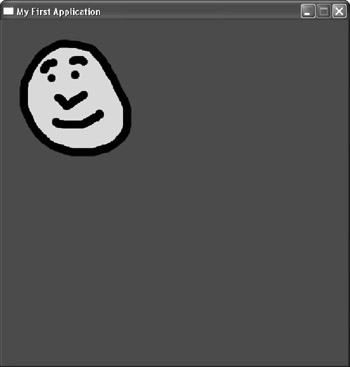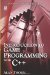# 16.3 Drawing Surfaces

## 16.3 Drawing Surfaces

As demonstrated, surfaces are drawn on the display via the draw method, and there are numerous versions of the draw method corresponding to the different ways a surface may be drawn. Following is a list of the draw methods for CL_Surface. These are the same methods used for sprites, which are discussed later in this chapter.

• void draw(floatx=0, floaty=0, CL_GraphicContext* context = 0);

• void draw(const CL_Rect& dest, CL_GraphicContext* context = 0);

• void draw(const CL_Rect& src, const CL_Rect& dest, CL_GraphicContext* context = 0);

• void draw(const CL_Surface_DrawParams1& params1, CL_GraphicContext* context = 0);

• void draw(const CL_Surface_DrawParams2& params2, CL_GraphicContext* context = 0);

Each of the draw methods contains an argument to a CL_Graphic-Context class which, as we shall see later in this chapter, allows a programmer to draw the contents of a surface on canvases other than the window. Surfaces can also be drawn on other surfaces and other kinds of objects.Figure 16.1

### 16.3.1 Drawing a Surface in a Rectangle

`      void draw(const CL_Rect& dest, CL_GraphicContext*      context = 0) `

A rectangle defines a rectangular area of space on the screen and can be described by a CL_Rect structure. Specifically, a rectangle can be defined by two points: the top-left corner and the bottom-right corner of the rectangle. These points correspond to the four members of CL_Rect: CL_Rect::left, CL_Rect::top, CL_Rect::right, and CL_Rect::bottom. So, a rectangle can be defined in code as follows:

`      CL_Rect Rect;      Rect.left = 0;      Rect.top = 0;      Rect.right = 500;      Rect.bottom = 500; `

Being able to define rectangles in this way means that not only can a surface be drawn at a specific x and y location, but it can be drawn to fit in a specified destination rectangle. In other words, the surface will be automatically stretched or squeezed to fit inside a specified rectangle. Consider the following Paint function:

`      void cClanLibApp::Paint()      {         CL_Display::clear(CL_Color(255,0,0));         CL_Rect Rect;         Rect.left = 0;         Rect.top = 0;         Rect.right = 500;         Rect.bottom = 500;         Surface->draw(Rect);      } `

### 16.3.2 Drawing a Rectangular Selection from a Surface in a Rectangle

`      void draw(const CL_Rect& src, const CL_Rect& dest,      CL_GraphicContext* context = 0); `

In addition to stretching the whole of a surface to fit inside a destination rectangle, it is also possible to select only a portion of the surface to stretch and draw in a destination rectangle. To select a portion of the surface, another rectangle is used. This rectangle specifies the source rectangle of the surface to be drawn; the origin (0,0) corresponds to the top-left corner of the surface, not the screen. Consider the following code:

`      void cClanLibApp::Paint()      {         CL_Display::clear(CL_Color(255,0,0));         CL_Rect Rect;         Rect.left = 0;         Rect.top = 0;         Rect.right = 500;         Rect.bottom = 500;         CL_Rect SrcRect;         SrcRect.left = 0;         SrcRect.top = 0;         SrcRect.right = 100;         SrcRect.bottom = 100;         Surface->draw(SrcRect, Rect);      } `

### 16.3.3 Drawing a Surface Repeatedly

Let's take the example of a surface loaded with a happy face image. We know how it can be drawn to the screen. If, however, we want two, three, or more happy face images drawn to the screen, we do not have to load additional surfaces with the same image. The same surface can be drawn many times per frame using any combination of the methods shown. Consider the following code:

`      void cClanLibApp::Paint()      {         CL_Display::clear(CL_Color(255,0,0));         for(int i=0; i<3; i++)         {            Surface->draw(30*i, 30*i);         }      } `Introduction to Game Programming with C++ (Wordware Game Developers Library)
ISBN: 1598220322
EAN: 2147483647
Year: 2007
Pages: 225
Authors: Alan Thorn

Similar book on Amazon

flylib.com © 2008-2017.
If you may any questions please contact us: flylib@qtcs.net